# What is the molarity of a solution containing 88 8 grams of solute in 1366 7...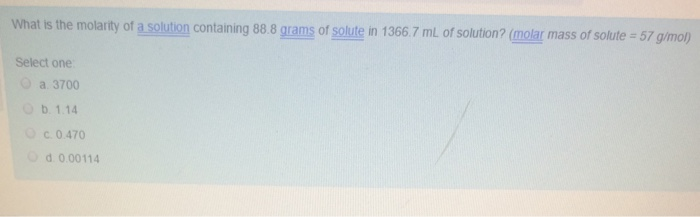What is the molarity of a solution containing 88 8 grams of solute in 1366 7 mL of solution? (molar mass of solute s = 57 g/mol)) Select one 3700 1.14 0.470 0.00114

Ans. Moles of solute = Mass/ Molar mass

= 88.8 g / (57 g/mol)

= 1.5579 mol

Volume of solution = 1366.7 mL = 1.3667 L                    ; [1 L = 1000 mL]

Now, molarity = Moles of solute / Volume of solution in liters

= 1.5579 mol / 1.3667 L

= 1.139 mol/ L

= 1.139 M = 1.14 M                                               ; [1 M = 1 mol/ L]

Thus, correct option is – b. 1.14 M

#### Earn Coin

Coins can be redeemed for fabulous gifts.

Similar Homework Help Questions
• ### 1a. An aqueous solution has a Molarity of 1.632 M. The density of the solution is...

1a. An aqueous solution has a Molarity of 1.632 M. The density of the solution is (1.150x10^0) g/mL and the solute has a molar mass of (1.33x10^2) g/mol. What is the molality of this solution? 1b. An aqueous solution has a mass percent of solute of 18.4%. The density of the solution is (1.400x10^0) g/mL and the solute has a molar mass of (1.77x10^2) g/mol. What is the molality of this solution? 1c. An aqueous solution has a molality of...

• ### n sucrose (molar mass 342.22 g) solution that 7. What is the molarity of a 1.45...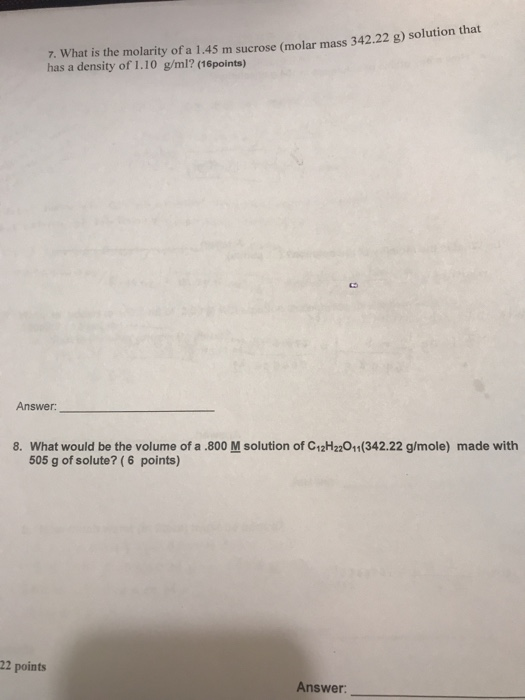n sucrose (molar mass 342.22 g) solution that 7. What is the molarity of a 1.45 m sucrose (molar mass 342.24 B has a density of 1.10 g/ml? (16 points) Answer: 8. What would be the volume of a .800 M solution of C12H22011(342.22 g/mole) made with 505 g of solute? (6 points) 22 points Answer:

• ### QUESTION 7 What is the molarity of a solution prepared by dissolving 1453 g of lactose...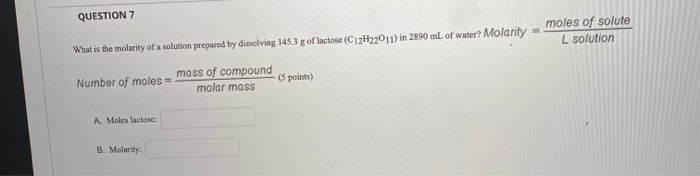QUESTION 7 What is the molarity of a solution prepared by dissolving 1453 g of lactose (C12H2011) in 2890 mL of water? Molanity- moles of solute L solution Number of moles mass of compound molar mass 5 points) A. Moles lactose B. Molarity:

• ### complete the table: Mass Mol Volume Solute Solute Solute Solution Molarity MgSO4 0.608g 24.0 mL NaOH...

complete the table: Mass Mol Volume Solute Solute Solute Solution Molarity MgSO4 0.608g 24.0 mL NaOH 105.0 mL 1.65 M CH3OH 14.5 g 0.550 M

• ### What molarity of sodium hydroxide solution is used to react 0.511 grams of KHP, if the...

What molarity of sodium hydroxide solution is used to react 0.511 grams of KHP, if the initial buret reading for the addition of sodium hyrdoxide is 0.4mL and the final reading is 12.7 mL? The molar mass of KHP is 204.2 g/mol. The reaction is KHP + NaOH --> KNaP +H2O

• ### Using molarity to calculate grams of solute. What is the concentration of ammonia in a solution...

Using molarity to calculate grams of solute. What is the concentration of ammonia in a solution made by dissolving 3.75 g of ammonia in 120.0 L of water?

• ### Complete the table Molarity Solute Mass of solute Moles of solute Volume of solution of solution...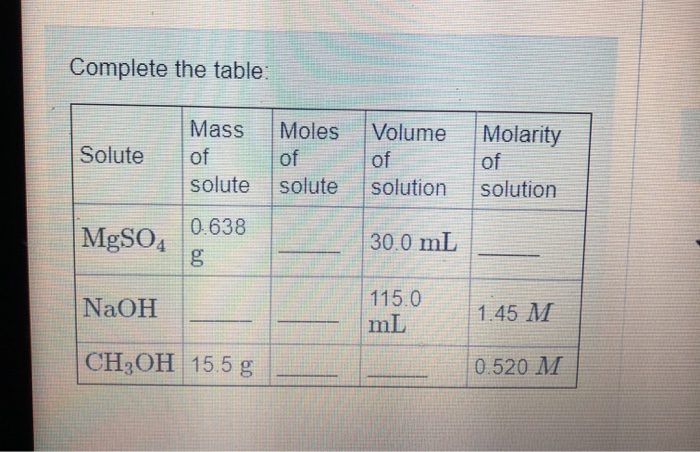Complete the table Molarity Solute Mass of solute Moles of solute Volume of solution of solution MgSO4 0.638 YLE 30.0 mL NaOH 115.0 ml 1.45 M CH3OH 15.5 g 0.520 M

• ### 8) What is the molarity (M) concentration of a solution when 18.37 grams of potasslum nitrite...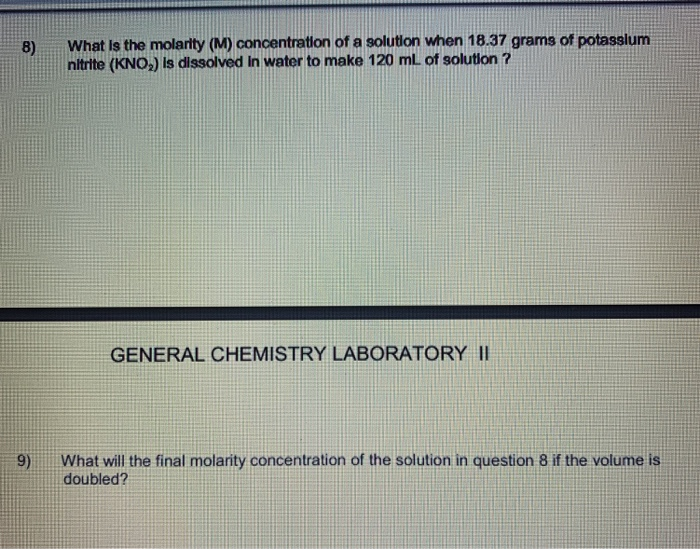8) What is the molarity (M) concentration of a solution when 18.37 grams of potasslum nitrite (KNO,) is dissolved in water to make 120 mL of solution ? GENERAL CHEMISTRY LABORATORY II 9) What will the final molarity concentration of the solution in question 8 if the volume is doubled? 10) How many milliliters of 0.153 M KMnO, solution are needed to react with 6.44 grams of iron(II) sulfate in aqueous solution? The balanced reaction is: 10 Feso, + 2...

• ### Grams solute 1.50 g NaCl Molarity/Titrations/stoichiometry Volume solution Molarity solution 100.0 mL 0.2104 M 250.0 mL...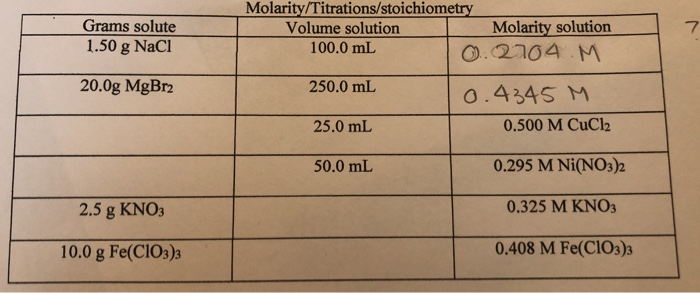Grams solute 1.50 g NaCl Molarity/Titrations/stoichiometry Volume solution Molarity solution 100.0 mL 0.2104 M 250.0 mL 0.4345 M 25.0 mL 0.500 M CuCl2 20.0g MgBr2 50.0 mL 0.295 M Ni(NO3)2 2.5 g KNO3 0.325 M KNO3 10.0 g Fe(ClO3)3 0.408 M Fe(ClO3)3

• ### What is the molarity of a solution that contains 50.0 grams of soduim hydroxide, NaOH in...

What is the molarity of a solution that contains 50.0 grams of soduim hydroxide, NaOH in 855 mL of solution? The molar mass of NaOH is 40.0 grams.

Free Homework Help App